Degrees, Radians, and Trig Angle Entries

Remember: The calculator defaults to Radian measure.

 Working with Degrees:

There are two ways to engage "Degrees" when working with the calculator:
1.  Set the MODE to Degree and all further calculations will be in degrees.
2.  If in Radian MODE, use the Degree Symbol (2nd APPS (Angle) and select #1 ° ).
The degree status will be applied to that one calculation only.  All other calculations

Find the radian measure of an angle whose measure is 500°.

 Method 1: (in Radian or Degree mode)  Convert by multiplying by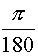. Mode set to Radian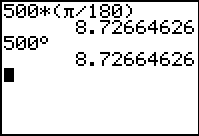Method 2:  (in Radian mode) With the mode set to Radian, type 500°.  Notice the degree symbol.  Hit Enter.

NOTE:  If in Degree mode, you can force an answer to radians by using
2nd APPS(Angle) #3 r.

 In Degree mode, but ......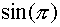gives the answer 0.0548036651 (WRONG!!!)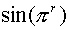gives the correct answer of 0.

Find the degree measure of an angle whose measure is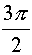Method 1: (in Radian or Degree  mode)  Convert by multiplying by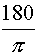. Mode set to Degree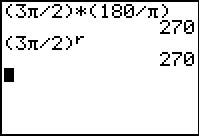Method 2:  (in Degree mode) In Degree mode, the r symbol can be used to convert radians to degrees.  The r is found 2nd APPS(Angle) #3 r.   With the mode set to Degree, type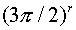Convert 57° 45' 17'' to decimal degrees:

In either Radian or Degree Mode:    Type 57° 45' 17'' and hit Enter.
° is under Angle (above APPS) #1
'  is under Angle (above APPS) #2
''  use ALPHA (green) key with the quote symbol above the + sign.

 Convert 57° 45' 17'' to radians:

With the mode set to Radian  Type 57° 45' 17'' °  and hit Enter.
** Note the use of the additional degree symbol at the end.  Without it, conversion would be to decimal degrees, even though the mode is set to radians.

 Convert 48.555° to degrees, minutes, seconds:

Type 48.555 ►DMS                          Answer:  48° 33' 18''
The ►DMS is #4 on the Angle menu (2nd APPS).  This function works even if Mode is set to Radian.

 Given cos A = .0258.  Find

With the mode set to Degree:  Type cos-1(.0258).  Hit Enter.
Engage ►DMS
(Be careful here to be in the correct mode!!)

 Find sin 57° 45' 17'':

Enter sin(57° 45' 17'' °).   This problem is clearly dealing with degrees.  The entry shown here works even if MODE is set to Radian.   Answer:  .8457717984
If MODE is set to Degree, the degree symbol at the end is not needed.

 Find cos(-250° 21'):

If MODE is set to Degree, the degree symbol at the end is not needed.

 Find sec(25° 40'):

There is no key for secant.  But, since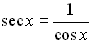, enter:  1/cos(25° 40' °) when in Radian mode.   If MODE is set to Degree, the degree symbol at the end is not needed.

 Find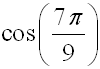:

Enter directly: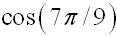when in Radian mode.    Answer:  -.7660444431
If in Degree mode,
enter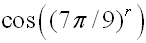.

 Find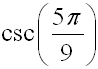:

There is no key for cosecant.  But, since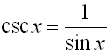, enter: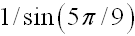when in Radian mode.   Answer:  1.015426612

In Degree mode, enter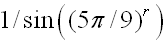.